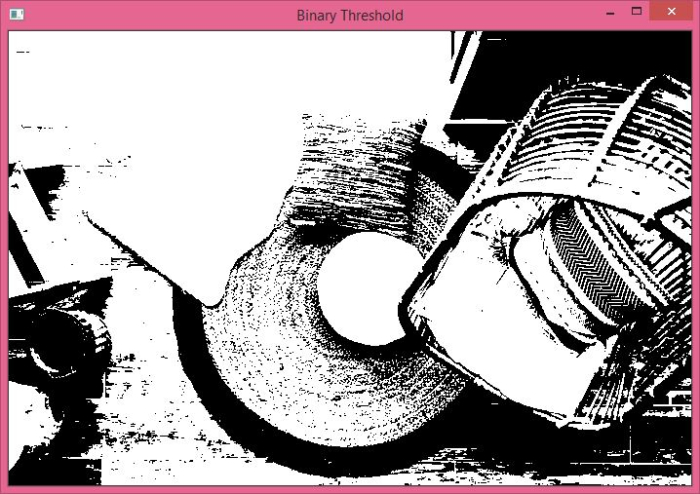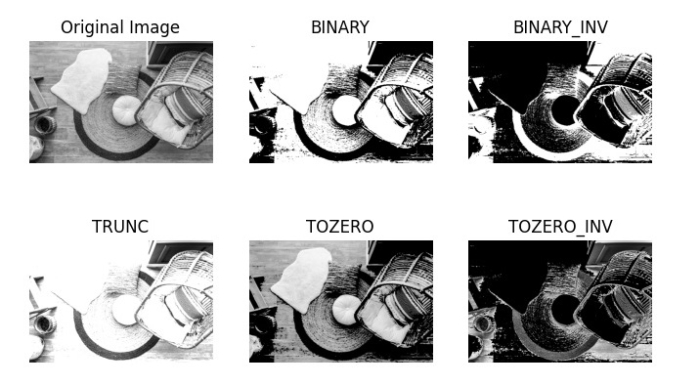# How to perform different simple thresholding of an image using Python OpenCV?

In simple thresholding, we define a threshold value and if a pixel value is greater than a threshold value, it is assigned a value (say 255), else it is assigned another value (say 0).

A simple thresholding can be applied using the function cv2.threshold(). It accepts four arguments− the source image, threshold value, the maxVal and the thresholding type.

OpenCV provides the following different types of thresholding −

• cv2.THRESH_BINARY − In this thresholding, pixel value more than the threshold value is assigned to 255 else assigned to 0.

• cv2.THRESH_BINARY_INV − It is the opposite case of cv2.THRESH_BINARY.

• cv2.THRESH_TRUNC − In this thresholding, pixel value more than the threshold assigned to threshold, other pixels remain the same.

• cv2.THRESH_TOZERO − In this thresholding, pixel value less than the threshold assigned to zero, other pixels remain the same.

• cv2.THRESH_TOZERO_INV − Opposite of cv2.THRESH_TOZERO.

## Syntax

cv2.threshold(img, thresh_val, max_val, thresh_type)


## Parameters

• img − Input gray scale image. It's a numpy.ndarray.

• thresh_val − Threshold value used to classify the pixel values. If the pixel value is higher than the threshold value, it is assigned one value else another value.

• max_val − Maximum value to be assigned to a pixel if the pixel value is more than (sometimes less than) the threshold value.

• thresh_type − The type of thresholding to be applied.

It returns the global threshold value and the threshold image.

Let's understand different simple thresholdings with the help of some Python examples.

## Input Image

We will use this image as the input file in the following examples.## Example 1

In this program, we apply Binary thresholding on the input image.

# Python program to illustrate
# a simple thresholding type on an image
# import required libraries
import cv2

# read the input image as a gray image

# applying binary thresholding technique on the input image
# all pixels value above 127 will be set to 255
_, thresh = cv2.threshold(img, 127, 255, cv2.THRESH_BINARY)

# display the image after applying binary thresholding
cv2.imshow('Binary Threshold', thresh)
cv2.waitKey(0)
cv2.destroyAllWindows()


## Output

When you run the above program, it will produce the following output −The above output shows the threshold image after applying Binary thresholding.

## Example 2

In this program, we will apply different Simple thresholding on the input image.

# Python program to illustrate
# simple thresholding type on an image
# import required libraries
import cv2
import matplotlib.pyplot as plt

# read the input image as a gray image
# applying different thresholding techniques on the input image
ret1, thresh1 = cv2.threshold(img, 127, 255, cv2.THRESH_BINARY)
ret2, thresh2 = cv2.threshold(img, 127, 255, cv2.THRESH_BINARY_INV)
ret3, thresh3 = cv2.threshold(img, 127, 255, cv2.THRESH_TRUNC)
ret4, thresh4 = cv2.threshold(img, 127, 255, cv2.THRESH_TOZERO)
ret5, thresh5 = cv2.threshold(img, 127, 255, cv2.THRESH_TOZERO_INV)

titles = ['Original Image','BINARY','BINARY_INV','TRUNC','TOZERO','TOZERO_INV']
images = [img,thresh1,thresh2,thresh3,thresh4,thresh5]

for i in range(6):
plt.subplot(2,3,i+1)
plt.imshow(images[i], 'gray')
plt.title(titles[i])
plt.axis("off")
plt.show()


## Output

When you run the above python program, it will produce the following output −The above output shows the different images after applying different simple thresholding.